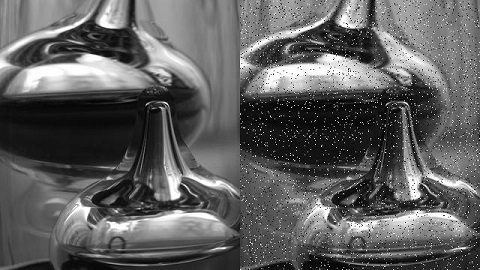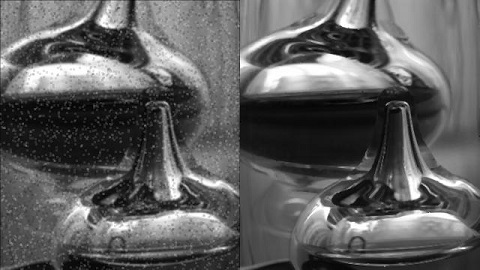# 数字图像处理/空间滤波/中值滤波

## 代码实现

10 * 10 的中值滤波器实现

``````import numpy as np
import PIL.Image
import scipy.misc
import scipy.ndimage

def convert_2d(r):
n = 10
s = scipy.ndimage.median_filter(r, (n, n))
return s.astype(np.uint8)

def convert_3d(r):
s_dsplit = []
for d in range(r.shape):
rr = r[:, :, d]
ss = convert_2d(rr)
s_dsplit.append(ss)
s = np.dstack(s_dsplit)
return s

im = PIL.Image.open('/img/jp.jpg')
im_mat = np.asarray(im)
im_converted_mat = convert_3d(im_mat)
im_converted = PIL.Image.fromarray(im_converted_mat)
im_converted.show()
``````

## 效果展示